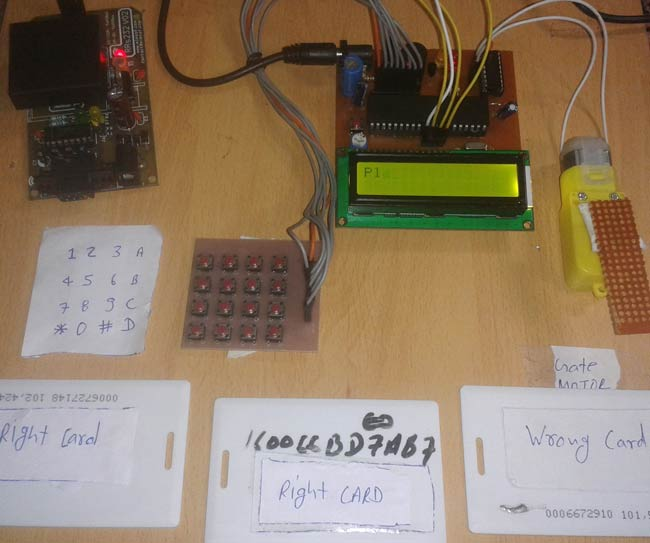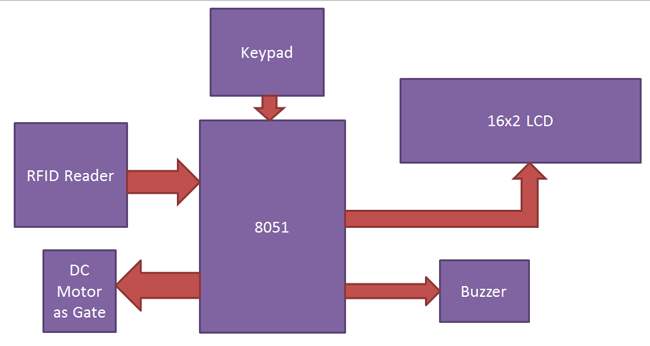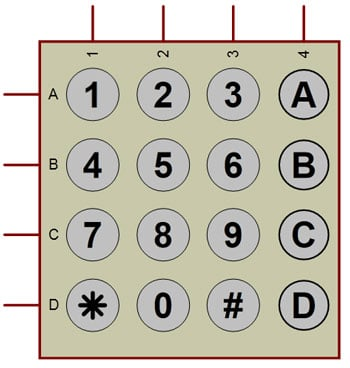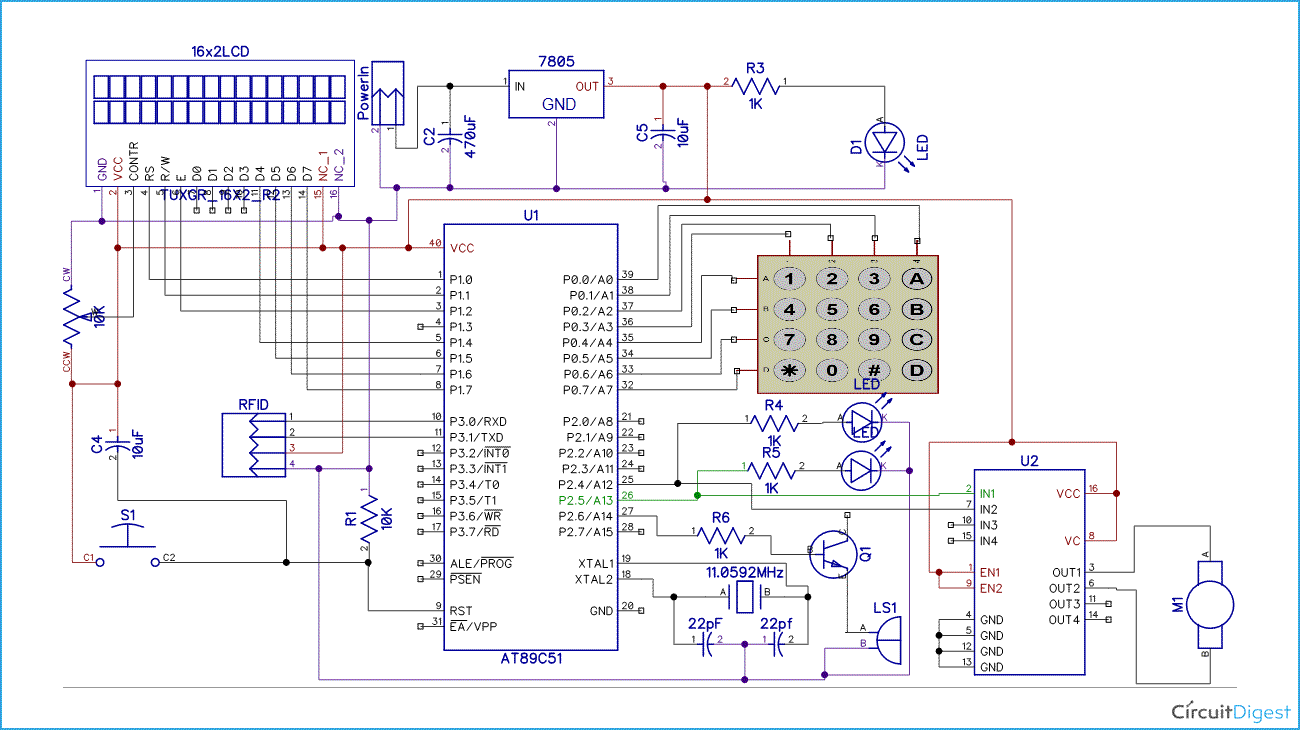# RFID and Keypad Based Security System using 8051 Microcontroller

Published  December 1, 2015   31
S
AuthorIn this project, we are going to develop an RFID and keypad based Security system. This project is implemented by using 8051 microcontroller. RFID Tecnology (Radio Frequency Identification and Detection) is commonly used in schools, colleges, office and stations for various purposes to automatically authenticate people with valid RFID tags. Here we will check the RFID tag, along with a password associated with the tag, to secure the system.

### Working

We can divide the complete security system into various sections -  Reader section, Keypad, Control section, Driver section and Display section. Working of the entire system and role of each section can be understood throgh the below block diagram.Reader Section: This section contains a RFID, which is an electronics device which has two parts - one is RFID Reader and other is RFID tag or Card. When we put RFID tag near the RFID reader it reads tag data serially. RFID tag which we have used here has 12 digit character code or serial number. This RFID is working at baud rate of 9600 bps.

Keypad: Here we have used a 4x4 matrix keypad for entering the password to the system. [Also check: 4x4 keypad interfacing with 8051]Control Section: 8051 microcontroller is used for controlling the complete process of this RFID based security system. Here by using 8051 we are receiving RFID data and sending status or messages to LCD.

Display Section: 6x2 LCD is used in this project for displaying messages on it. Here you can see the tutorial: LCD interfacing with 8051 microcontroller

Driver Section: This section has a motor driver L293D for opening gate and a buzzer with a BC547 NPN transistor for indications.

When a person put his RFID tag to RFID reader then RFID reads tag’s data and send it to 8051 microcontroller and then microcontroller compares this data with pre-defined data. If data is matched with predefined data then microcontroller ask for password and after entering password microcontroller compare password with predefined password. If password match gate will open otherwise LCD show Access denied and buzzer start beeping for sometime.

### Circuit Diagram and ExplanationAs shown in above RFID security system circuit diagram, 16x2 LCD is connected in four bit mode with microcontroller. LCD’s RS, RW and EN pins are directly connected at PORT 1 pin number P1.0, P1.1 and P1.2. D4, D5, D6 and D7 pins of LCD is directly connected at pin P1.4, P1.5, P1.6 and P1.7 of port 1. Motor driver is connected at PORT pin number P2.4 and P2.5. And buzzer is connected at P2.6 at PORT2. And keypad is connected at PORT0. Keypad row are connected at P0.4 – P0.7 and Columns are connected at P0.0 – P0.3.

### Program Explanation

While programming the 8051 microcontroller for RFID based security system, first of all we include header files and defines input and output pin and variables.

```#include<reg51.h>
#include<string.h>
#include<stdio.h>
#define lcdport P1

sbit rs=P1^0;
sbit rw=P1^1;
sbit en=P1^2;

sbit m1=P2^4;
sbit m2=P2^5;
sbit buzzer=P3^1;

char i,rx_data;

char rfid,ch=0;

char pass;```

Then define pins for keypad module.

```sbit col1=P0^0;
sbit col2=P0^1;
sbit col3=P0^2;
sbit col4=P0^3;
sbit row1=P0^4;
sbit row2=P0^5;
sbit row3=P0^6;
sbit row4=P0^7;```

After this we have created a function for delay.

``` void delay(int itime)
{
int i,j;
for(i=0;i<itime;i++)
for(j=0;j<1275;j++);
}```

Then we make some function for LCD and initialize lcd function,

```void lcd_init(void)
{
lcdcmd(0x02);
lcdcmd(0x28);
lcdcmd(0x0e);
lcdcmd(0x01);
}```

Here we have some function that we have used in our program. In this we have configured 9600bps baud rate at 11.0592MHz Crystal Frequency, and fuction for receiving we are monitoring the SBUF register for receiving data.

```void uart_init()
{
TMOD=0x20;
SCON=0x50;
TH1=0xfd;
TR1=1;
}
char rxdata()
{
while(!RI);
ch=SBUF;
RI=0;
return ch;
}```

After this in main program we have initialized lcd and Uart and then we read the output of RFID when any tag is bring on it. We store this string in an array and then match with predefind array data. And then match the password.

```        if(strncmp(rfid,"160066A5EC39",12)==0)
{
if(strncmp(pass,"4201",4)==0)
{
accept();
lcdcmd(1);
lcdstring("Access Granted ");
lcdcmd(0xc0);```

If match occurs then controller opens the gate, otherwise buzzer starts and LCD shows invalid card.

Code

#include<reg51.h>
#include<string.h>
#include<stdio.h>
#define lcdport P1

sbit col1=P0^0;
sbit col2=P0^1;
sbit col3=P0^2;
sbit col4=P0^3;
sbit row1=P0^4;
sbit row2=P0^5;
sbit row3=P0^6;
sbit row4=P0^7;

sbit rs=P1^0;
sbit rw=P1^1;
sbit en=P1^2;

sbit m1=P2^4;
sbit m2=P2^5;
sbit buzzer=P3^1;

char i,rx_data;

char rfid,ch=0;

char pass;

void delay(int itime)
{
int i,j;
for(i=0;i<itime;i++)
for(j=0;j<1275;j++);
}

void daten()
{
rs=1;
rw=0;
en=1;
delay(5);
en=0;
}

void lcddata(unsigned char ch)
{
lcdport=ch & 0xf0;
daten();
lcdport=(ch<<4) & 0xf0;
daten();
}

void cmden(void)
{
rs=0;
en=1;
delay(5);
en=0;
}

void lcdcmd(unsigned char ch)
{
lcdport=ch & 0xf0;
cmden();
lcdport=(ch<<4) & 0xf0;
cmden();
}

void lcdstring(char *str)
{
while(*str)
{
lcddata(*str);
str++;
}
}

void lcd_init(void)
{
lcdcmd(0x02);
lcdcmd(0x28);
lcdcmd(0x0e);
lcdcmd(0x01);
}

void uart_init()
{
TMOD=0x20;
SCON=0x50;
TH1=0xfd;
TR1=1;
}
char rxdata()
{
while(!RI);
ch=SBUF;
RI=0;
return ch;
}

{
lcdcmd(1);
lcdstring("Enter Ur Passkey");
lcdcmd(0xc0);
i=0;
while(i<4)
{
col1=0;
col2=col3=col4=1;
if(!row1)
{
lcddata('1');
pass[i++]='1';
while(!row1);
}

else if(!row2)
{
lcddata('4');
pass[i++]='4';
while(!row2);
}

else if(!row3)
{
lcddata('7');
pass[i++]='7';
while(!row3);
}

else if(!row4)
{
lcddata('*');
pass[i++]='*';
while(!row4);
}

col2=0;
col1=col3=col4=1;
if(!row1)
{
lcddata('2');
pass[i++]='2';
while(!row1);
}

else if(!row2)
{
lcddata('5');
pass[i++]='5';
while(!row2);
}

else if(!row3)
{
lcddata('8');
pass[i++]='8';
while(!row3);
}

else if(!row4)
{
lcddata('0');
pass[i++]='0';
while(!row4);
}

col3=0;
col1=col2=col4=1;
if(!row1)
{
lcddata('3');
pass[i++]='3';
while(!row1);
}

else if(!row2)
{
lcddata('6');
pass[i++]='6';
while(!row2);
}

else if(!row3)
{
lcddata('9');
pass[i++]='9';
while(!row3);
}

else if(!row4)
{
lcddata('#');
pass[i++]='#';
while(!row4);
}

col4=0;
col1=col3=col2=1;
if(!row1)
{
lcddata('A');
pass[i++]='A';
while(!row1);
}

else if(!row2)
{
lcddata('B');
pass[i++]='B';
while(!row2);
}

else if(!row3)
{
lcddata('C');
pass[i++]='C';
while(!row3);
}

else if(!row4)
{
lcddata('D');
pass[i++]='D';
while(!row4);
}
}

}

void accept()
{
lcdcmd(1);
lcdstring("Welcome");
lcdcmd(192);
delay(200);
}

void wrong()
{
buzzer=0;
lcdcmd(1);
lcdstring("Wrong Passkey");
lcdcmd(192);
lcdstring("PLZ Try Again");
delay(200);
buzzer=1;
}

void main()
{
buzzer=1;
uart_init();
lcd_init();
lcdstring("  RFID Based    ");
lcdcmd(0xc0);
lcdstring("Security system ");
delay(400);
while(1)
{
lcdcmd(1);
lcdcmd(0xc0);
i=0;
for(i=0;i<12;i++)
rfid[i]=rxdata();
rfid[i]='\0';
lcdcmd(1);
lcdcmd(0xc0);
for(i=0;i<12;i++)
lcddata(rfid[i]);
delay(100);
if(strncmp(rfid,"160066A5EC39",12)==0)
{
if(strncmp(pass,"4201",4)==0)
{
accept();
lcdcmd(1);
lcdstring("Access Granted ");
lcdcmd(0xc0);
lcdstring("Person1");
m1=1;
m2=0;
delay(300);
m1=0;
m2=0;
delay(200);
m1=0;
m2=1;
delay(300);
m1=0;
m2=0;
}
else
wrong();
}

else if(strncmp(rfid,"160066BD7AB7",12)==0)
{
if(strncmp(pass,"4202",4)==0)
{
accept();
lcdcmd(1);
lcdstring("Access Granted ");
lcdcmd(0xc0);
lcdstring("Person2");
m1=1;
m2=0;
delay(300);
m1=0;
m2=0;
delay(200);
m1=0;
m2=1;
delay(300);
m1=0;
m2=0;
}
else
wrong();
}

else if(strncmp(rfid,"160066203060",12)==0)
{
if(strncmp(pass,"4203",4)==0)
{
accept();
lcdcmd(1);
lcdstring("Access Granted ");
lcdcmd(0xc0);
lcdstring("Person3");
m1=1;
m2=0;
delay(300);
m1=0;
m2=0;
delay(200);
m1=0;
m2=1;
delay(300);
m1=0;
m2=0;
}
else
wrong();
}

else
{
lcdcmd(1);
buzzer=0;
delay(300);
buzzer=1;
}
}
}

Video

Tags

Submitted by QAMAR SHAIKH on Wed, 12/23/2015 - 07:06

### RFID

GR8 WORK ,KEEP IT UP

Submitted by Saeed Mahdavi Asl on Sun, 06/26/2016 - 13:40

thanks alot

### you can convert above C code

you can convert above C code into hex file using keil uvisio software.

Submitted by lee on Mon, 09/19/2016 - 20:47

### hello :) can i know what name

hello :) can i know what name of component label as U2?

### ok TQ =) if I using proteus

ok TQ =) if I using proteus to draw and run this circuit, what should I type in proteus library to find RFID?

Submitted by MD on Thu, 09/29/2016 - 15:29

### code not correct

your program have errors so plzz send original code

Submitted by Susmita Banerjee on Wed, 10/19/2016 - 22:51

### Sir can you provide the hex

Sir can you provide the hex code . Is this code can be compiled in any c complier or any specific compiler is there?

Submitted by Yi on Thu, 11/03/2016 - 23:30

### If i wanted to add more tags

If i wanted to add more tags and passwords how will i do that? and how many persons can you store?

### Currently we have hard coded

Currently we have hard coded the the RFID tags IDs so you cant add on run time, but you can alter ID in code for your card. Or you can modify the code to add IDs on run time and share here for the community.

Submitted by joe on Mon, 11/07/2016 - 05:11

### What RFID module is that

What RFID module is that where can i get it from? also tags?

### Its 'Em18 Rfid Card Reader

Its 'Em18 Rfid Card Reader Module', you can buy it online or from Local electronic components shop.

Submitted by PRADEEP KUMAR on Tue, 12/13/2016 - 12:16

Dear Abi
I am Pradeepkumar working in Salem Steel Plant as Sr Mgr Electrical. I started my Carrier in Electronic Lab and having more interest in doing projects as hobby. Earlier I did in 8085 ..then small projects in 89c51 ...now started learning Arduino. I used to invest money to purchase sensors and processor and doing myself in House. But main technical supports are from NET, like you people. But now-a-days people are crazy to put their project as youtube without code and circuit diagram. So some time people like me are blinking.
But you have given the project in detail. It is very nice of you. By your this type of helping, you can develop many electronic engineers in India. let me once again Congrads you abi. All the best. Pl send your mail id if u like to have contact to my mail id []. Thanks Abi

Submitted by Papai Karmakar on Sun, 12/25/2016 - 20:42

### Access card

How to set pass key in the access card ?

Submitted by KA on Fri, 02/03/2017 - 12:16

### Is this a correct code or is

Is this a correct code or is it having any errors.?? If so please send me the correct code... If possible hex file

Submitted by lee on Tue, 02/07/2017 - 19:51

### in power section, what type

in power section, what type of power source you use? is it 9v battery?

Submitted by pooja on Fri, 02/17/2017 - 18:33

### Sir,

Sir,
I tried the same code given above, but could not understand the flaws.... the rfid reader reads the tag code... but does not display the rfid code in lcd... it shows junk characters..... would you mind helping me out... that what would be reason behind this issue

Submitted by Nikesh juware on Fri, 03/31/2017 - 21:28

### rfid& microcontrolle

microcontroller size is 4k and program size is 4.59 so the microcontroller does not work?

Submitted by murtaza on Sun, 09/03/2017 - 14:55

### I'm doing this project and I

I'm doing this project and I want a schematic file to run a proteus simulation.Can u please email me the file.

Submitted by MANUEL on Wed, 12/13/2017 - 20:29

### RFID MODULE

How can I find that RFID module? what´s its name?

Submitted by mobile on Tue, 12/19/2017 - 17:03

Submitted by Amoolya on Sat, 04/07/2018 - 16:50

what is the conponent specification used as rfid reader?

Submitted by Kumar on Wed, 04/18/2018 - 19:31

### eeprom based writing 4 Mobile no. And read it -

Sir I need a code for storing 4 mobile no. After loading the hex file ,the no.has editable via key pad

### can u send circuit diagram of rfid

Can u please send the rfid pins connected to the circuit iam using RC522 a bit confusing with the pins.Since there are 8 pins in it.

Thank you

hello, I had made this project but the display is not showing output , it only glows when power is given. Can you please tell me the solution for that as soon as possible